Open Access Publications from the University of California

### Recent Work

This is the website for papers published by the Center for Pervasive Communications and Computing at the University of California, Irvine.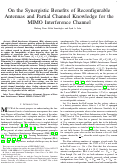## On the Synergistic Benefits of Reconfigurable Antennas and Partial Channel Knowledge for the MIMO Interference Channel

(2021)

Blind Interference Alignment (BIA) schemes create and exploit channel coherence patterns without the knowledge of channel realizations at transmitters, while beamforming schemes rely primarily on channel knowledge available to the transmit- ters without regard to channel coherence patterns. In order to explore the compatibility of these disparate ideas and the possibility of synergistic gains, this work studies the Degrees of Freedom (DoF) of the 2-user (?1 × ?1)(?2 × ?2) Multiple- Input Multiple-Output (MIMO) Interference Channel (IC) where Transmitter 1 is equipped with reconfigurable antennas and has no channel knowledge, while Transmitter 2 has partial channel knowledge but no reconfigurable antennas. Taking a fundamental dimensional analysis perspective, the main question is to identify which antenna configurations allow synergistic DoF gains. The main results of this work are two-fold. The first result identifies antenna configurations where both reconfigurable antennas and partial channel knowledge are individually beneficial, as those where ?1 < ?1 < min(?2,?2). The second result shows that synergistic gains exist in each of these settings, over the best known solutions that rely on either reconfigurable antennas or partial channel knowledge alone. Coding schemes that jointly exploit partial channel knowledge and reconfigurable antennas emerge as a byproduct of the analysis.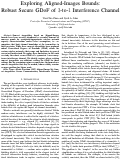## Exploring Aligned-Images Bounds:Robust Secure GDoF of 3-to-1 Interference Channel

(2020)

Sum-set inequalities based on Aligned-Images bounds have been recently introduced as essential elements of converse proofs for asymptotic/approximate wireless network capacity characterizations under robust assumptions, i.e., as- sumptions that limit channel knowledge at the transmitters to finite precision. While these sum-set inequalities have produced robust Generalized Degrees of Freedom (GDoF) results for various wireless networks, their scope and limitations in general are not well understood. To explore these limitations, in this work we study the robust secure GDoF of a symmetric 3-user many- to-one interference channel. We identify regimes where existing sum-set inequalities are sufficient, settling the GDoF for those settings. For the remaining regime we conjecture the form of new sum-set inequalities that may be needed, whose validity remains an open problem for future work.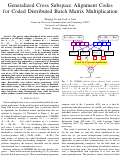(2019)

The goal of coded distributed batch matrix multiplication  is to efficiently multiply  L instances of \lambda x \kappa matrices, A=(A_1, ..., A_L)$, with L instances of \kappa x \mu matrices B=(B_1,..., B_L), by distributing the computation across S servers, such that the response from any R servers (R is called the recovery threshold) is sufficient to compute the L matrix products, AB=(A_1B_1, A_2B_2, ..., A_LB_L). Existing solutions either compute each$A_lB_lone at a time by partitioning individual matrices and coding across these partitions, or rely only on batch processing, i.e., coding across the batch of matrices without any matrix partitioning. The state-of-art for matrix-partitioning and batch processing approaches is represented by Entangled Polynomial Codes (EP codes), and Lagrange Coded Computing (LCC), respectively. In order to combine the benefits of the two approaches, we propose Generalized Cross-Subspace Alignment Codes (GCSA codes) that unify, generalize and improve upon the state of art. GCSA codes bridge the two extremes by efficiently combining both matrix-partitioning and batch processing, and offer flexibility in how much of each approach is used. Both EP codes and LCC codes can be recovered as special cases of GCSA codes. Remarkably, even without matrix partitioning, GCSA codes demonstrate an advantage over LCC codes in download-constrained settings. This is due to cross-subspace alignment, characterized by a Cauchy-Vandermonde code structure that aligns interference along Vandermonde terms, while the desired matrix products remain resolvable along Cauchy terms.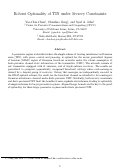## Robust Optimality of TIN under Secrecy Constraints (2019) A parameter regime is identified where the simple scheme of treating interference as Gaussian noise (TIN), with power control and jamming, is optimal for the secure generalized degrees of freedom (GDoF) region of Gaussian broadcast networks under the robust assumption of finite-precision channel state information at the transmitter (CSIT). The network consists of one transmitter equipped with K antennas, and K single-antenna receivers. The results are generalized to groupcast (equivalently, compound broadcast) settings where each message is desired by a disjoint group of receivers. Noting that messages are independently encoded in the GDoF-optimal scheme, the result for the broadcast channel is extended to its counterpart Gaussian interference channel under finite precision CSIT. Evidently, both secrecy constraints and finite precision CSIT limit the benefits of more sophisticated schemes, leading to optimality of simpler schemes for larger parameter regimes. Aligned Image bounds are the key to the proof of optimality for these larger parameter regimes under finite precision CSIT.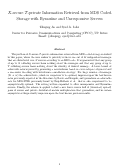##X$-secure$T$-private Information Retrieval from MDS Coded Storage with Byzantine and Unresponsive Servers (2019) The problem of$X$-secure$T$-private information retrieval from MDS coded storage is studied in this paper, where the user wishes to privately retrieve one out of$K$independent messages that are distributed over$N$servers according to an MDS code. It is guaranteed that any group of up to$X$colluding servers learn nothing about the messages and that any group of up to$Tcolluding servers learn nothing about the identity of desired message. A lower bound of achievable rates is proved by presenting a novel scheme based on \emph{cross-subspace alignment} and a successive decoding with interference cancellation strategy. For large number of messages(K\rightarrow\infty)the achieved rate, which we conjecture to be optimal, improves upon the best known rates previously reported in the literature by Raviv and Karpuk, and generalizes an achievable rate for MDS-TPIR previously found by Freij-Hollanti et al. that is also conjectured to be asymptotically optimal. The setting is then expanded to allow unresponsive and Byzantine servers. Finally, the scheme is applied to find a new lower convex hull of (download, upload) pairs of secure and private distributed matrix multiplication that generalizes, and in certain asymptotic settings strictly improves upon the best known previous results.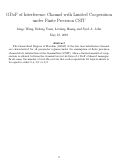## GDoF of Interference Channel with Limited Cooperation under Finite Precision CSIT (2019) The Generalized Degrees of Freedom (GDoF) of the two user interference channel are characterized for all parameter regimes under the assumption of finite precision channel state information at the transmitters (CSIT), when a limited amount of cooperation is allowed between the transmitters in the form of π DoF of shared messages. In all cases, the number of over- the-air bits that each cooperation bit buys is shown to be equal to either 0, 1, 1/2 or 1/3.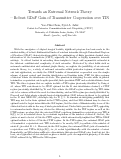## Towards an Extremal Network Theory – Robust GDoF Gain of Transmitter Cooperation over TIN (2019) With the emergence of aligned images bounds, significant progress has been made in the understanding of robust fundamental limits of wireless networks through Generalized Degrees of Freedom (GDoF) characterizations under the assumption of finite precision channel state information at the transmitters (CSIT), especially for smaller or highly symmetric network settings. A critical barrier in extending these insights to larger and asymmetric networks is the inherent combinatorial complexity of such networks. Motivated by other fields such as extremal combinatorics and extremal graph theory, we explore the possibility of an extremal network theory, i.e., a study of extremal networks within particular regimes of interest. As our test application, we study the GDoF benefits of transmitter cooperation over the simple scheme of power control and treating interference as Gaussian noise (TIN) for three regimes of interest where the interference is weak. The question is intriguing because while in general transmitter cooperation can be quite powerful, finite precision CSIT and weak interference favor TIN. The three regimes that we explore include a TIN regime previously identified by Geng et al. where TIN was shown to be GDoF optimal for theK$user interference channel, a CTIN regime previously identified by Yi and Caire where the GDoF region achievable by TIN turns out to be convex without the need for time-sharing, and an SLS regime previously identified by Davoodi and Jafar where a simple layered superposition (SLS) scheme is shown to be optimal in the$K$user MISO BC, although only for$K\leq 3$. It remains an intriguing possibility that TIN may not be far from optimal in the CTIN regime, and that SLS schemes may be close to optimal even for larger networks in the SLS regime, but the curse of dimensionality is one of the obstacles that stands in the way of such generalizations. Under finite precision CSIT, appealing to extremal network theory we obtain the following results. In the TIN regime as well as the CTIN regime, we show that the extremal GDoF gain from transmitter cooperation over TIN is$\Theta(1)$, i.e., it is bounded above by a constant regardless of the number of users$K$. In fact, the gain is at most a factor of$2$in the CTIN regime, which automatically implies that TIN is GDoF optimal within a factor of$2$in the CTIN regime. In the TIN regime, the extremal GDoF gain of transmitter cooperation over TIN is shown to be exactly$50\%$, regardless of the number of users$K$, provided$K>1$. However, in the SLS regime, the extremal GDoF gain of transmitter cooperation over TIN is$\Theta(\log_2(K))$, i.e., it scales logarithmically with the number of users. Remarkably, an SLS scheme suffices to demonstrate this extremal GDoF gain. Last but not the least, as a byproduct of our analysis we prove a useful cyclic decomposition property of the sum GDoF achievable by TIN in the SLS regime.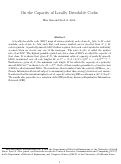## On the Capacity of Locally Decodable Codes (2018) A locally decodable code (LDC) maps$K$source symbols, each of size$L_w$bits, to$M$coded symbols, each of size$L_x$bits, such that each source symbol can be decoded from$N \leq M$coded symbols. A perfectly smooth LDC further requires that each coded symbol is uniformly accessed when we decode any one of the messages. The ratio$L_w/L_x$is called the symbol rate of an LDC. The highest possible symbol rate for a class of LDCs is called the capacity of that class. It is shown that given$K, N$, the maximum value of capacity of perfectly smooth LDCs, maximized over all code lengths$M$, is$C^*=N\left(1+1/N+1/N^2+\cdots+1/N^{K-1}\right)^{-1}$. Furthermore, given$K, N$, the minimum code length$M$for which the capacity of a perfectly smooth LDC is$C^*$is shown to be$M = N^K$. Both of these results generalize to a broader class of LDCs, called universal LDCs. The results are then translated into the context of PIR$_{\max}$, i.e., Private Information Retrieval subject to maximum (rather than average) download cost metric. It is shown that the minimum upload cost of capacity achieving PIR$_{\max}$schemes is$(K-1)\log N\$. The results also generalize to a variation of the PIR problem, known as Repudiative Information Retrieval (RIR).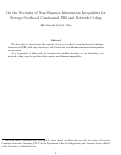## On the Necessity of Non-Shannon Information Inequalities for Storage Overhead Constrained PIR and Network Coding

(2018)

We show that to characterize the capacity of storage overhead constrained private information retrieval (PIR) with only 2 messages, and 2 databases, non-Shannon information inequalities are necessary. As a by-product of this result, we construct the smallest instance, to our knowledge, of a network coding capacity problem that requires non-Shannon inequalities.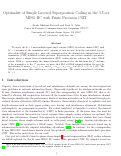## Optimality of Simple Layered Superposition Coding in the 3 User MISO BC with Finite Precision CSIT

(2018)

We study the 3 user multiple input single output (MISO) broadcast channel (BC) with 3 antennas at the transmitter and 1 antenna at each receiver, from the generalized degrees of freedom (GDoF) perspective, under the assumption that the channel state information at the transmitter (CSIT) is limited to finite precision. In particular, our goal is to identify a parameter regime where a simple layered superposition (SLS) coding scheme achieves the entire GDoF region. With αij representing the channel strength parameter for the link from the jth antenna of the transmitter to the ith receiver, we prove that SLS is GDoF optimal without the need for time-sharing if max(αji,αij) ≤ αii and αki + αij ≤ αii + αkj for all i,j,k ∈ . The GDoF region under this condition is a convex polyhedron.